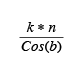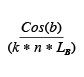## Grating Dispersion

Diffraction gratings are used to split light into separate wavelengths, or to disperse the light.

Angular dispersion is a correlation between a change in the diffraction angle and a small change in wavelength. Angular dispersion iswhere

b = Diffracted Angle
k = Diffracted Order
n = Groove Density

Linear dispersion will vary with the distance from the grating to the detector. Linear dispersion iswhere

b = Diffracted Angle
k = Diffracted Order
n = Groove Density
LB = Distance from Grating to Detector